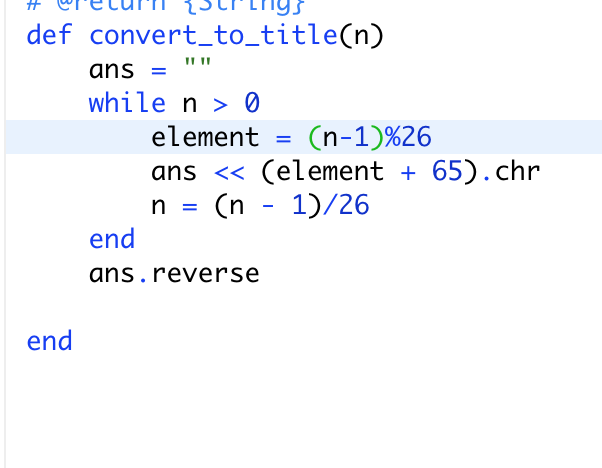# Confused on why we do n-1

#1

I saw the explanation below but it still isn’t making much sense to me.

#2

Because 1 should map to 0 -> ‘A’+0
and 27 should map to 00 and so on.
It is an artefact of 1 based indexing.
The fact that you are dividing by 26 should make it obvious that 26 itself is not ‘A’+0 but ‘A’+25.
Also the result of division starts with 1 again.

#3But why are we mapping 1 to 0?
Why can’t we do n%27 or keep the indexes as 1-26 and only add 64?
I understand why we divide by 26 at the end, but I’m still confused as to why we need to do n-1 for this division.

#4

Last step has n-1 as imagine a number 26^2 * 3+26^1 * 7+26. For the next step in the loop, we have to eliminate the last digit i.e., 26. If we do n=n/26, we’ll get 26 * 3+7+1. So, you see we still have 1 at the end. If we do n=(n-1)/26, we’ll get 26 * 3+7+0. This eliminates the last digit.

#5

Just consider case of A = 26 .you wont be getting “Z” as output .